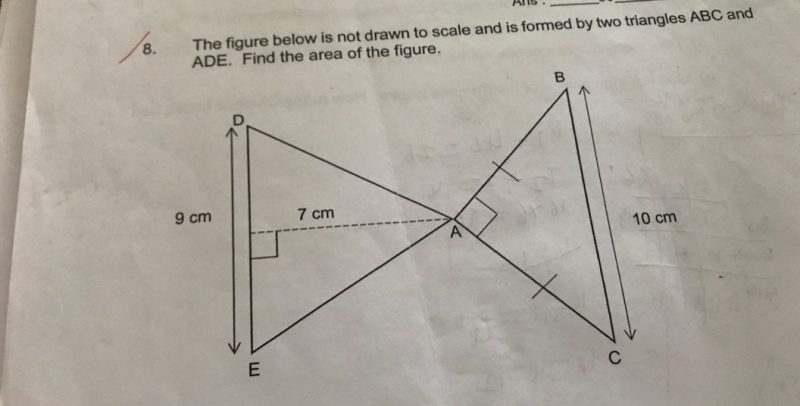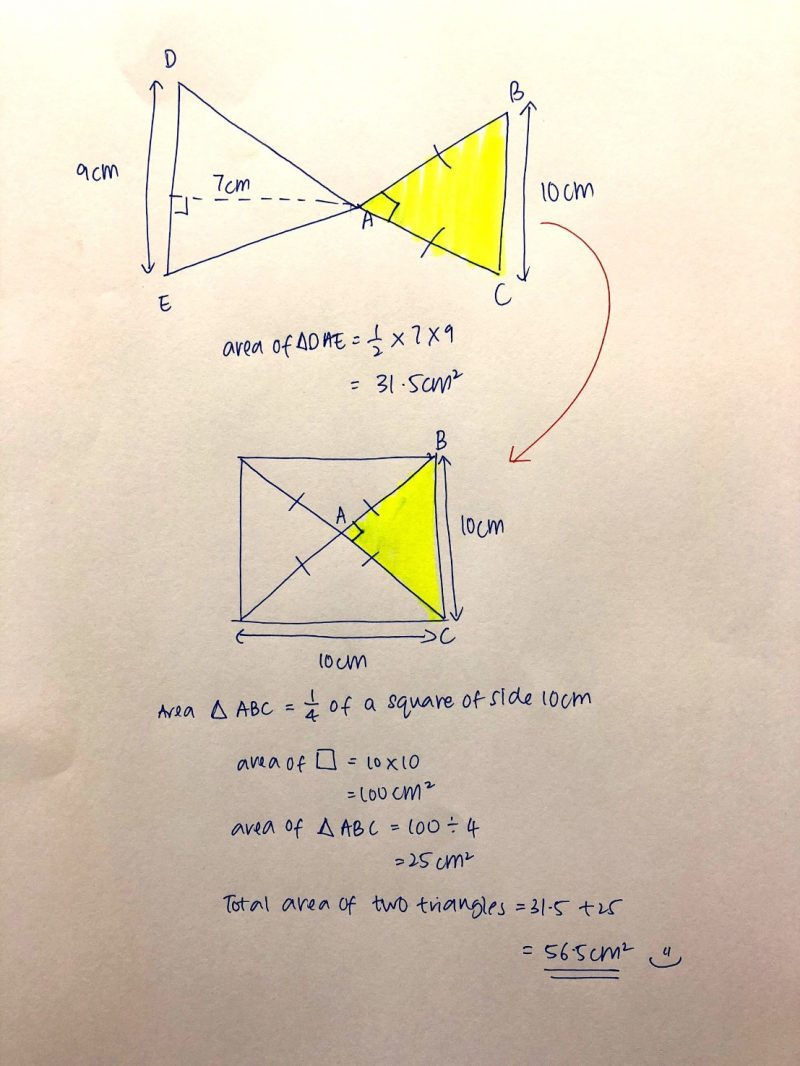# QuestionHi,

Thank you0 Replies 0 Likes

The mid point of DE is 4.5cm.

Area of ADE = 4.5 * 7 = 31.5cm square

Triangle ABC is also half a square as two side of same length with angle A at 90 degree.

10cm is the diagonal length of the square.

From A to mid point of BC, is also half of the diagonal length.

10/2 = 5cm (This is A to midpoint of BC)

Area of ABC = 5 * 5 = 25cm square

Total area of figure = 25+31.5 = 56.5cm square

Steps

Half of DE = 9 / 2 = 4.5cm

Area of ADE = 4.5 * 7 = 31.5cm square

A to mid of BC = 10 / 2 = 5cm

Area of ABC = 5 * 5 = 25cm square

Total area of figure = 25 + 31.5 = 56.5cm square

0 Replies 0 Likes